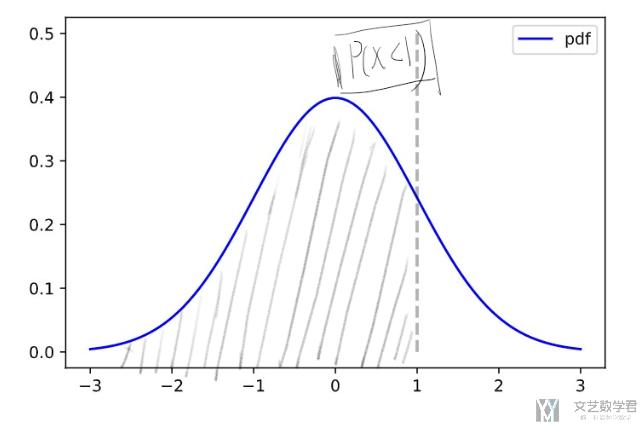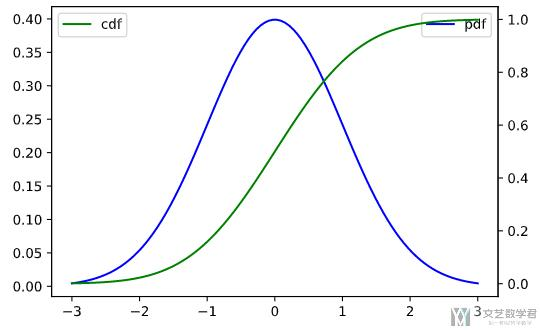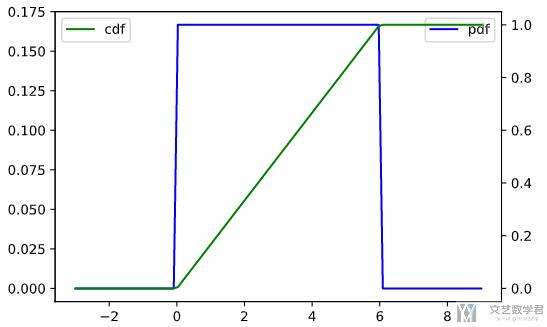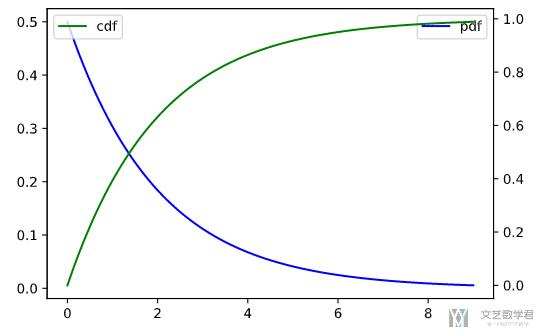# matplotlib 可视化概率密度函数(pdf)和累计分布函数(cdf)2021年2月17日07:11:32

1 2476字阅读8分15秒

## PDF 和 CDF 的介绍

1. from scipy.stats import norm
2. mean = 0
3. std = 1
4. norm.pdf(0, mean, std)
5. """
6. 0.3989422804014327
7. """

1. mean = 0
2. std = 1
3. norm.cdf(1, mean, std)
4. """
5. 0.8413447460685429
6. """## 使用 Matplotlib 可视化 pdf 和 cdf

### 正态分布

1. # 绘制 pdf 和 cdf 的函数
2. mean = 0
3. std = 1
4. fig = plt.figure()
6. x_index =  np.linspace(-3, 3, 100)
7. data_pdf_data = [norm.pdf(i, mean, std) for i in x_index] # pdf 数据
8. data_cdf_data = [norm.cdf(i, mean, std) for i in x_index] # cdf 数据
9. ax.plot(x_index, data_pdf_data, color='b', label='pdf')
10. ax_twin = ax.twinx() # 次坐标
11. ax_twin.plot(x_index, data_cdf_data, color='g', label='cdf')
12. ax.legend()
13. ax_twin.legend()### 均匀分布

1. from scipy.stats import norm, uniform
2. # ########################
3. # 绘制均匀分布的 pdf 和 cdf 的函数
4. # ########################
5. loc = 0
6. scale = 6 # [loc, loc + scale]
7. fig = plt.figure()
9. x_index =  np.linspace(-3, 9, 100)
10. data_pdf_data = [uniform.pdf(i, loc, scale) for i in x_index] # pdf 数据
11. data_cdf_data = [uniform.cdf(i, loc, scale) for i in x_index] # cdf 数据
12. ax.plot(x_index, data_pdf_data, color='b', label='pdf')
13. ax_twin = ax.twinx() # 次坐标
14. ax_twin.plot(x_index, data_cdf_data, color='g', label='cdf')
15. ax.legend()
16. ax_twin.legend()### 指数分布

1. from scipy.stats import norm, uniform, expon
2. # 绘制 pdf 和 cdf 的函数
3. loc = 0
4. scale = 2 # pdf = lambda * exp(-lambda * x), scale = 1 / lambda
5. fig = plt.figure()
7. x_index =  np.linspace(0, 9, 100)
8. data_pdf_data = [expon.pdf(i, loc, scale) for i in x_index] # pdf 数据
9. data_cdf_data = [expon.cdf(i, loc, scale) for i in x_index] # cdf 数据
10. ax.plot(x_index, data_pdf_data, color='b', label='pdf')
11. ax_twin = ax.twinx() # 次坐标
12. ax_twin.plot(x_index, data_cdf_data, color='g', label='cdf')
13. ax.legend()
14. ax_twin.legend()• 微信公众号
• 关注微信公众号
•• QQ群
• 我们的QQ群号
•• 本文由 发表于 2021年2月17日07:11:32
• 转载请务必保留本文链接：https://mathpretty.com/13537.html